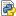Navigation

##### Personal tools
You are here: Home / delphiBindingCustom.py

# delphiBindingCustom.py

Example for a customized Delphi Binding Energy workflow.delphiBinding.py — Python Source, 3 KB (3461 bytes)

## File contents

```#!/usr/bin/env python
from Biskit import *
import Biskit.Dock as D
import numpy as N
import Biskit.gnuplot as G
import Biskit.tools as T
import sys
import Biskit.tools as T
import copy

## take input file from first command line argument
f_in = sys.argv
base = T.stripFilename( f_in )

## take complex separation as second argument
separation = 18.
if len(sys.argv) > 2:
separation = float(sys.argv)

f_ocom = base + '_delphi.complex'
f_out= base + '_delphi_energies.out'

def report( com ):
t = '%4s : %4s %7s %7s %7s %7s %7s' %\
('Rec','Lig','dG(kT)','solv','coul','ions','dG(kcal/mol)\n')

s = '%(rec)4s : %(lig)4s %(dG_kt)7.2f %(solv)7.2f %(coul)7.2f %(ions)7.2f %(dG_kcal)7.2f\n'

d = copy.copy(com['dG_delphi'])
d.update( {'rec':com.rec_model.pdbCode, 'lig':com.lig_model.pdbCode} )

r = t
r += s % d
return r

def separateComplex( com, separation ):
"""Create a gap of <distance> A between receptor and ligand"""
cr = com.rec().centerOfMass()
cl = com.lig().centerOfMass()
v0  = cr - cl  # distance vector
d0  = N.sqrt( N.sum( v0**2 ) )  # distance
delta = (v0 / d0) * separation  # multiply unity vector

matrix = com.ligandMatrix
matrix[:,3] = N.concatenate( (-delta, [1.]) ) # substract separation vector
com.setLigMatrix( matrix )

## load structure into PDBModel object
m = PDBModel( f_in )

## remove double-occupancies and non-standard residues, identify Cys-Cys bridges
## cap the first 4 chains with ACE (identify chain breaks by residue-distance)
clean = PDBCleaner( m, verbose=True )
m = clean.process( amber=True )
m = clean.capTerminals( capN=[0,1,2,3], breaks=True ) ## manually cap free N

## call reduce to optimize H-bond network and add hydrogens
red = Reduce( m, debug=False, autocap=False, verbose=True )
m = red.run()
m.xplor2amber()

## now remove ACE caps as they are not charged anyway and can have clashes
m = m.compress( N.array(m['residue_name']) != 'ACE' )

## map partial atomic charges from AMBER prep files into structure
ac = AtomCharger( verbose=True )
ac.charge( m )

## report missing partial charges (any residues with partial total charge)
residues = m.resModels()
qres = [ N.sum( x['partial_charge'] ) for x in residues ]

print 'Overall charge of system: ', N.sum( m['partial_charge'] )

for i, q in enumerate( qres ):
if q < -0.05 or q > 0.05:
if round( q, 1 ) != 1.0 and round( q, 1 ) != -1.0:
print '%2i %3s %2i %+3.1f' % (i, residues[i]['residue_name'], \
residues[i]['residue_number'], q)

print "Entering Delphi calculation"

## create Dock.Complex objects from first and second chain of PDBModel m
com = D.Complex( m.takeChains(  ), m.takeChains(  ) )
com.rec_model.pdbCode = 'Che'
com.lig_model.pdbCode = 'Cit'

## create a gap by shifting the ligand away from the receptor
if separation != 0.:
separateComplex( com, separation )

## the actual calculation
dg = D.DelphiBindingEnergy( com, protonate=False, addcharge=False,
scale=2.3, verbose=True, debug=False )
r = dg.run()

print "Saving result complex to ", f_ocom
T.dump( dg.delphicom, f_ocom )

print "Saving result complex to ", f_ocom
T.dump( dg.delphicom, f_ocom )

print "Final Result"
print "============"
print report( dg.delphicom )

f = open( f_out, 'w' )
f.write( report( dg.delphicom ) )
f.close()
print "energy values written to ", f_out
```